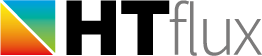# Ψg – ground contact calculations

The following pages will show you how to calculate the so called Ψg values. These linear thermal transmittances values are used to precisely calculate to energy loss to the ground. As always the calculation of the Ψ value is necessary to turn the simplified (surface based) U-value calculation of the buildings energy loss into a precise calculation. Omitting the calculation of these thermal bridging coefficients can therefore lead to significantly false energy calculations.

It is important to understand that these Ψg values for the “ground-floor junction” do not just describe the additional heat loss of the actual slab edge. Ψg values are used to correct the overall error made by using the simplified U-value calculations for the ground floor. Therefor it is important to note that a certain width of the floor slab has to be used for the simulation of the floor slab or basement. The Ψg value will only be valid for this actual width.

Unfortunately, various methods are used to carry out the Ψg calculation. Since HTflux is used world-wide and by various types of experts (building scientists, passive house planers, architects, …) it covers all calculation methods.

Depending on your calculation requirements you will have to pick the method suitable for your needs. Here is an overview of the main calculation methods (click on the titles for the detailed description):

### Ψg-calculation with slab on or near the grade level according to ISO 10211 and ISO 13370

The method involves the calculation of a Ug-value for the floor slab according to ISO13370. In HTflux the U-value and Heatflux-tool is used for the measurements and the PSIg-tool is being applied for the actual calculation of the Ψg value.

### Ψg-calculation with slab on or near the grade level according to ISO 10211 – Option B

The so called “option B” of ISO 10211 allows a simplified calculation of a Ψg value with the actual use of the Ug value defined in ISO 13370. In HTflux the U-value and Heatflux-tool is used for the necessary measurements and the PSIg-tool is being applied for the calculation of the Ψg value.

### Ψg-calculation with slab below grade level (or heated basement) using ISO 10211 and ISO 13370

This option has to be used when the slab is significantly below ground level. Similar to the Ug-value used for the floor slab a special Ubw-value has to be calculated for the wall below ground level. In HTflux the U-value and Heatflux-tool is used for the measurements and the PSIg-tool is being applied for the calculation.

### Ψg-calculation using boundary conditions as ground (ENEV)

This method is often allowed as a simplified calculation option. Like in the German ENVE calculations. The calculation with HTflux is very easy as only the automatic PSI-tool is required.

### Ψg-calculation using a soil block and boundary condition as ground

Apart from the ISO calculation this method is also used by some passive house designers. The calculation with HTflux is very easy as only the automatic PSI-tool is required for this purpose.